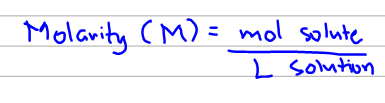# Problem: Determine the molarity of each of the following solutions:(c) 20.54 g of Al(NO3)3 in 1575 mL of solution

###### FREE Expert Solution

Molarity is given by the formula below:So first we have to calculate for the moles of solute. We do that by dimensional analysis using molar mass:

93% (146 ratings)###### Problem Details

Determine the molarity of each of the following solutions:

(c) 20.54 g of Al(NO3)3 in 1575 mL of solution